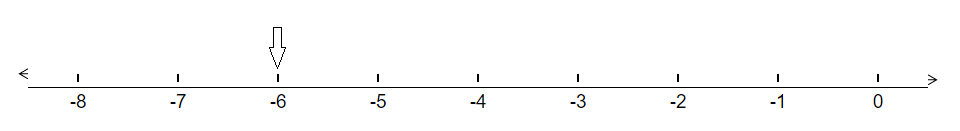# Represent the following numbers on a number line :(a) $+ 5$ (b) $‚Äì 10$ (c) $+ 8$(d) $‚Äì 1$ (e) $‚Äì 6$

To do:

We have to represent the given numbers on a number line.

Solution:

(a) $+5$ is 5 units right of $0$.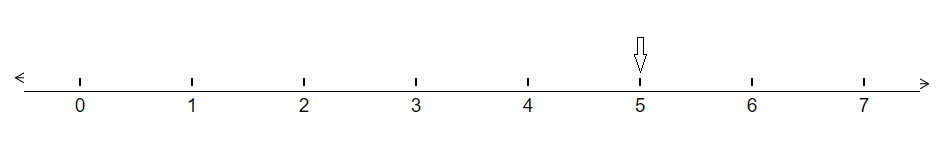(b) $-10$ is 10 units left of $0$.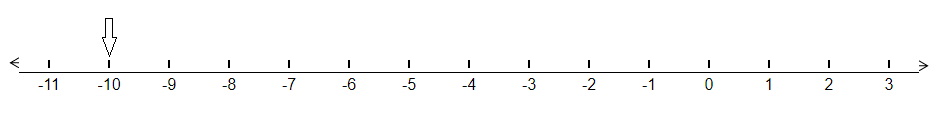(c) $+8$ is 8 units right of $0$.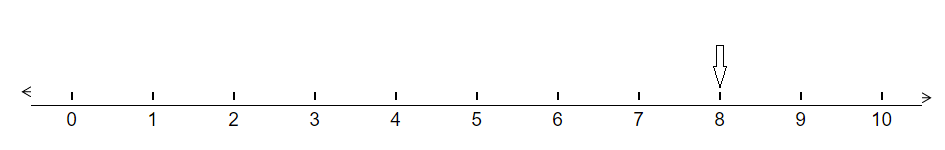(d) $-1$ is 1 unit left of $0$.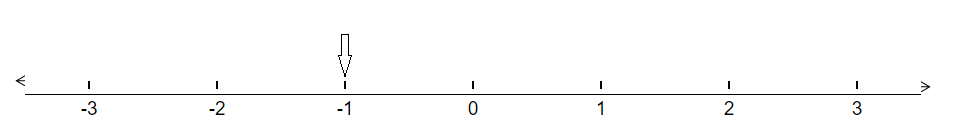(e) $-6$ is 6 units left of $0$.